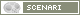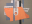OpenMusic DocumentationOM 6.6 User Manual > Basic Tools > Curves and Functions > BPF / BPC
page précédentepage suivante

2D Objects : BPF / BPC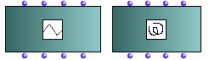The BPF and BPC boxes OM offers two types of objects for the manipulation of 2D curves :the BPF and BPC objects – Break Point Function and Break-Point Curve .

Properties

Inputs and Outputs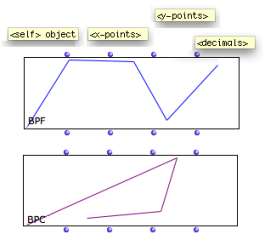BPF and BPC objects have four inputs and outputs :"self" : as in every object box, represents the object instance itself – BPF or BPC" x-points" : abscissa, a list of positive or negative numbers " y –points" : ordinates, a list of positive or negative numbers"decimals" : a number of decimals representing the approximation or step value of the points.

Creating BPF or BPC in a Patch

 The BPF or BPC points can be set by connecting lists of values to the "x-points" and "y-points" inputs, or with the BPF/BPC editor.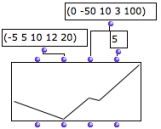X-Points Order in BPFs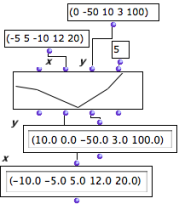In a BPF, x-points must be in a strictly increasing order. If the list is not ordered correctly, the points will be created from the y-points values and sorted automatically before the actual BPF is created.
Incomplete Coordinates Lists
 If the x or y list is shorter than the other, the last interval in the shorter list is repeated until the right number of points is reached.For instance, this allows to create a BPF with a list of y-points and a periodic x interval.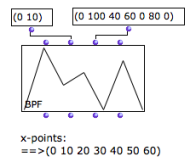Decimals and BPF/BPC Precision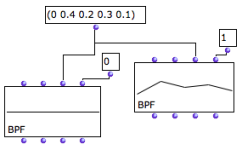Rounding or increasing precision of the point coordinates with "decimals". The " decimals" input determines the number of decimals per coordinates , that is, the precision of the curve. Coordinates are therefore rounded according to the number of decimals.If "decimals"= 0, all coordinates are rounded to the closest integer.
1. Break Point Function

A "break-point" function is a function defined by a finite number of points (x,y).

page précédentepage suivante
A propos...(c) Ircam - Centre Pompidou